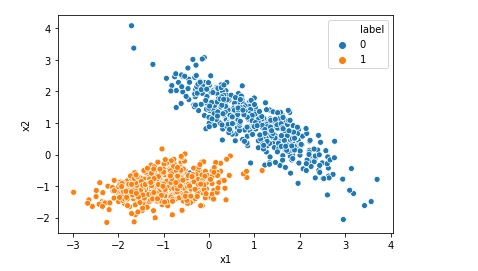# Cross-Validation in Machine Learning and K-fold Cross-Validation using Sklearn

Cross-Validation in Machine Learning for example K-fold Cross-Validation is a short video to describe what is Cross-Validation in Machine Learning, why do we need to do cross-validation, and how to do it using sklearn. https://youtu.be/9YqhJUSWSj8 Cross-Validation in Machine Learning and K-fold Cross-Validation using Sklearn Happy Learning !!# Print ROC AUC Receiver Operating Characteristic Area Under Curve

The receiver operating characteristic area under curve is a way to measure the performance of a classification model, may be created using algorithms like Logistic Regression. ROC-AUC is basically a graph where we plot true positive rate on y-axis and false positive rate on x-axis. If a model is good the AUC will be close to 1. Area … Continue reading Print ROC AUC Receiver Operating Characteristic Area Under Curve# Creating Synthetic Data for Logistic Regression

Many a times we want to implement Logistic Regression on certain data but we do not find that kind of data online. In that case we can generate a synthetic data for our problem. In this post we will see how to generate a typical synthetic data for a simple Logistic Regression. Import the required … Continue reading Creating Synthetic Data for Logistic Regression# Visualize and Print Confusion Matrix

In many cases you would like to print the confusion matrix in a better format and look and feel than what is provided by scikit learn by default. The default look when printing confusion matrix using scikit learn scikit-learn default confusion matrix print However in many cases you may like to print the confusion matrix … Continue reading Visualize and Print Confusion Matrix# What is Root Mean Squared Error or RMSE

Root mean squared error or RMSE is a measure of the difference between actual values and predicted values of a machine learning model  like Linear Regression. Root mean squared error is a measure of how well the machine learning model can perform. The lower the RMSE, the better the model. RMSE is always positive, and … Continue reading What is Root Mean Squared Error or RMSE# What is R Squared for Linear Regression

For Linear Regression, R-squared is a statistical term which indicates how close the data are to the fitted regression line. R-Squared is also known as coefficient of determination. R-squared = Explained variation in data / Total variation in data R-squared = 1 - (RSS/TSS) RSS = Sum of squares of difference between predicted value and … Continue reading What is R Squared for Linear Regression# Implementing Logistic Regression in 10 lines in Python

Logistic Regression is one of the most popular Machine Learning algorithm used for the classification problems. It should be noted that though there is a regression word in the name of the algorithm Logistic Regression, it is used for classification problems. A use case of Logistic regression could be, based on the symptoms for a disease that a patient has Logistic … Continue reading Implementing Logistic Regression in 10 lines in Python